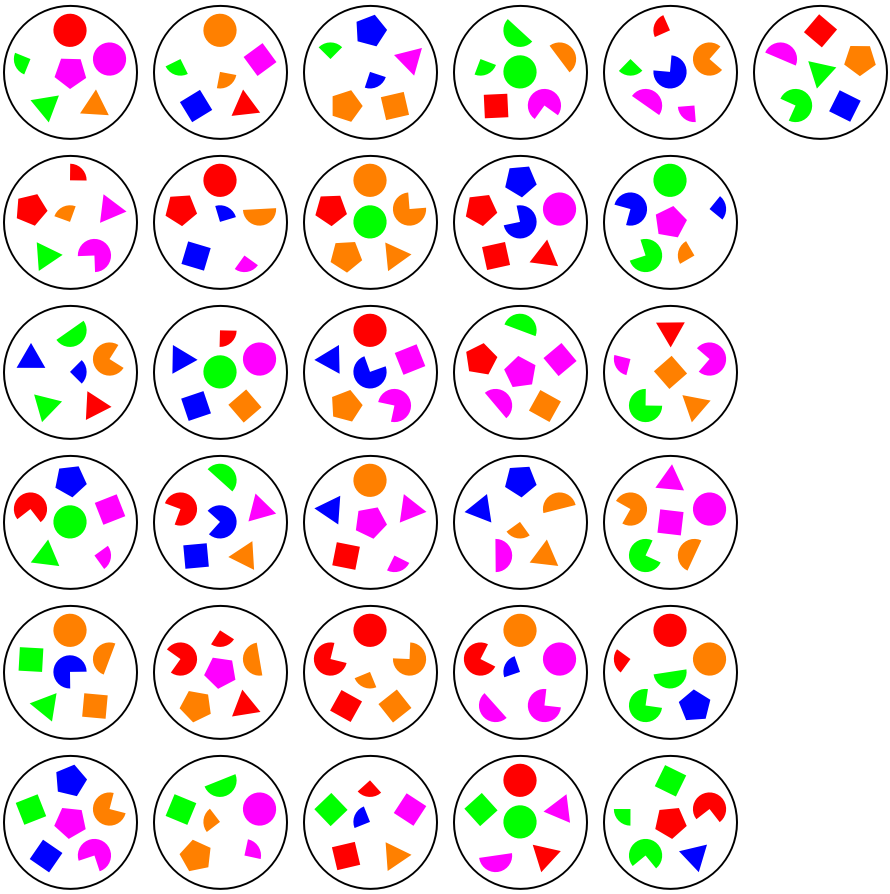Function Repository Resource:

DobbleSets

Generate a set of integers that can create Dobble cards

Contributed by: Sander Huisman
 ResourceFunction["DobbleSets"][n] generates n2-n+1 sets each with n numbers where each pair of sets only has a single number in common.

Details

Dobble is also known as Spot It! in some parts of the world.
n should be an integer.
n-1 should be prime or n should be 2, 5, 9 or 10.
ResourceFunction["DobbleSets"][n] creates n2-n+1 sets each with n numbers containing the number 1 through n2-n+1.

Examples

Basic Examples (2)

Create a small Dobble set:

 In:=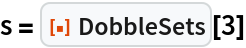Out=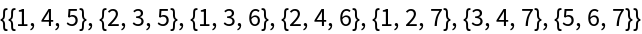Verify that each pair of sets has a single number as overlap:

 In:=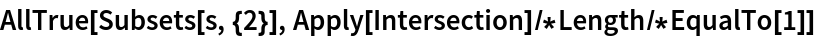Out=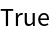Scope (2)

Large sets can be created for primes + 1:

 In:=Out=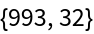Verify that each pair has a single number as overlap:

 In:=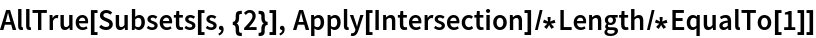Out=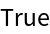Possible Issues (1)

Not all sizes are possible or are unknown:

 In:=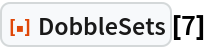Out=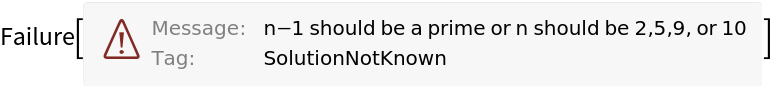Neat Examples (1)

Generate a set of Dobble-inspired cards where each 2 cards have only 1 symbol in common:

 In:=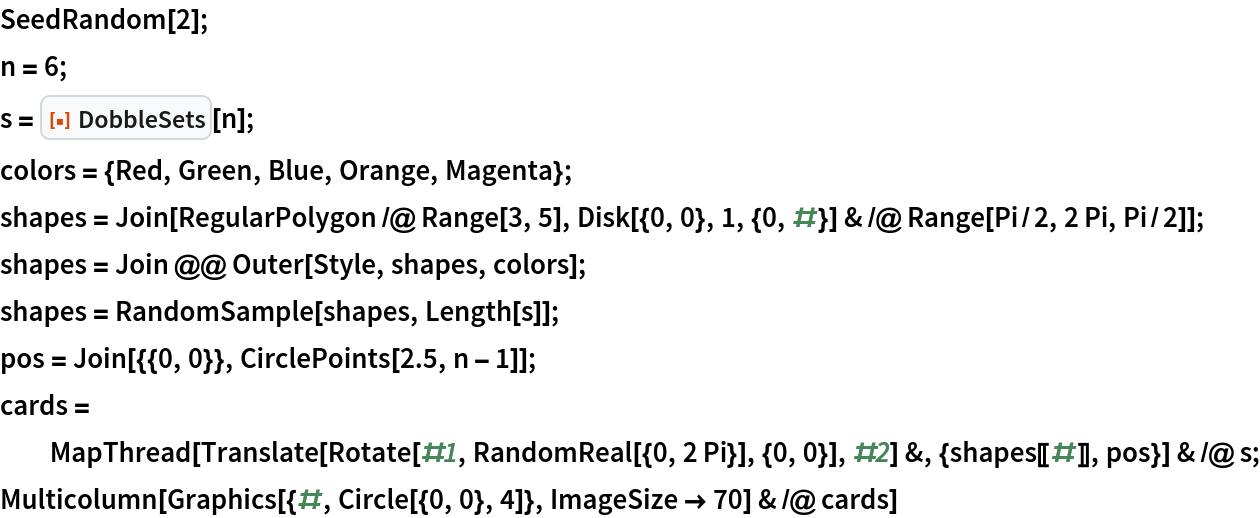Out=